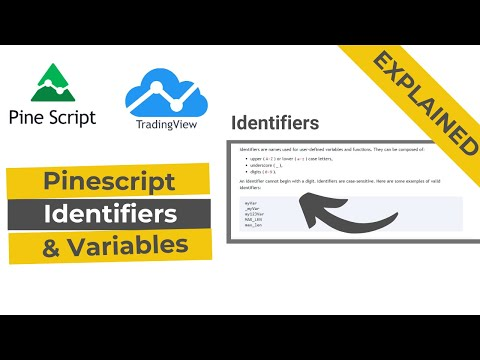Pine Script Tutorial

# Pine script variables and identifier

After learning the basic syntax of the pine script, this article is about variables. Variables are used to store data. Like in terms of forex, if you want to store a value of a currency pair then you will assign that specific value to a custom variable. So that a custom variable can be used throughout the program without calculating a value again and again.
In a programming language, variables are of vital importance. Variables represent data. During program execution, variables are replaced with real data automatically. So instead of entering data again and again within a program, variables are used.

## What is Identifier

The names which are used for custom variables and functions are called identifiers. A user can store information within names and it can be used throughout the program by calling identifiers.

This is very important because whenever you want the indicator to show a price or line on the chart then you will also use the identifier to store data. You will come to know in the upcoming articles.

The name of identifiers must be unique. It can have the following characteristics

• Identifiers can be named using small letters (a to z)
• Capital letters can be used (A to Z)
• Digits (0 to 9)
• Underscore (_)
• the identifier must not begin with a digit
• The identifier name is case sensitive. It means you can use the same small letter and capital letter but cannot use it more than one time.

For example
A= 1
a= 1
fibonacci_61 = 61.8
Fibonacci _50= 50.0### Multi-line statement in pine script

It’s mean one statement can be written in multiple lines. But the number of spaces must not be multiple of 4. Code will give an error if the number of spaces is 4 or multiple of 4. Pine script has a built-in function of the vertical line after every 4 spaces. So you don’t need to remember the number of spaces.
Check the code below

``````//@version=4
a= 1
+
1
plot(a)``````

This code will give you the following error. this error is due to the fact that there are 4 number spaces in the 5th line before digit 1. as I already have told you the number of spaces must not be multiple of 4. also look at the red circle that shows the verticle line. this verticle line reminds you about 4 spaces.

``Syntax error at input 'end of line without line continuation'``

### What type of information (variables) can be stored in identifiers?

• Identifiers can store the following type of information
• Integers (345, 6, 888)
• Floats (1.345, 3.543, number that has a decimal place)
• Strings (” hello world”, text or value within single or double quotes)